Скачать презентацию SOLVE EACH EQUATION OR INEQUALITY 1 x

d4b4f79770781bec385f856ee8c94f01.ppt

• Количество слайдов: 16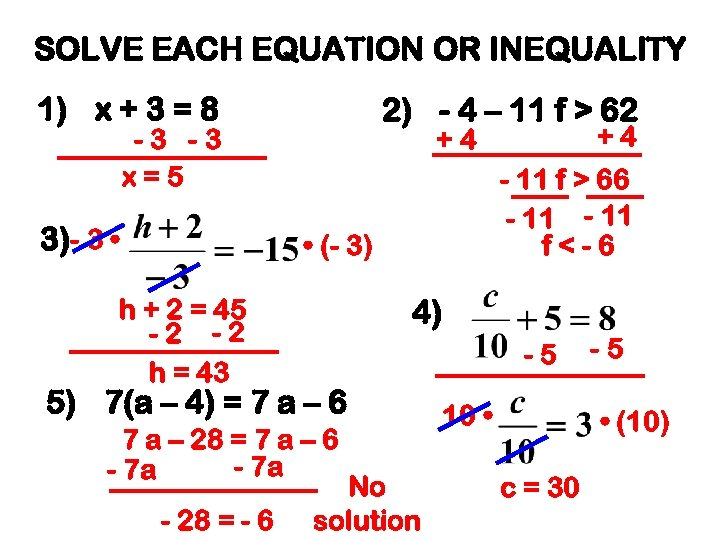SOLVE EACH EQUATION OR INEQUALITY 1) x + 3 = 8 2) - 4 – 11 f > 62 -3 -3 x=5 3)- 3 • - 11 f > 66 - 11 f<-6 • (- 3) h + 2 = 45 -2 -2 h = 43 4) -5 5) 7(a – 4) = 7 a – 6 7 a – 28 = 7 a – 6 - 7 a - 28 = - 6 +4 +4 No solution 10 • -5 • (10) c = 30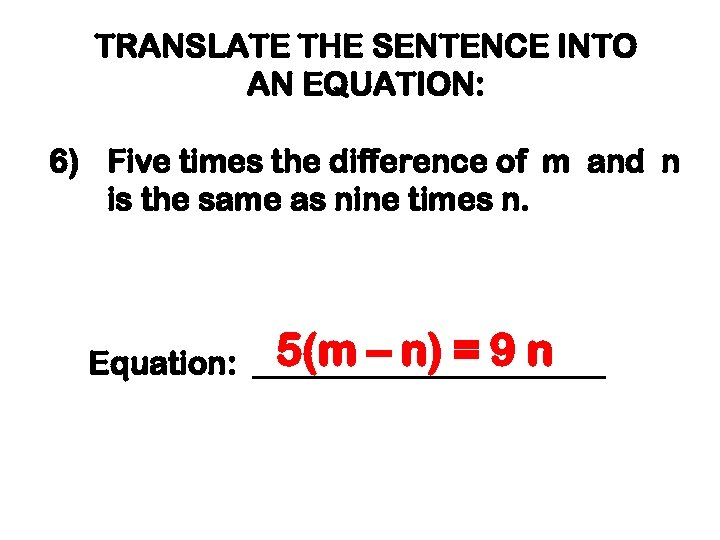TRANSLATE THE SENTENCE INTO AN EQUATION: 6) Five times the difference of m and n is the same as nine times n. 5(m – n) = 9 n Equation: ___________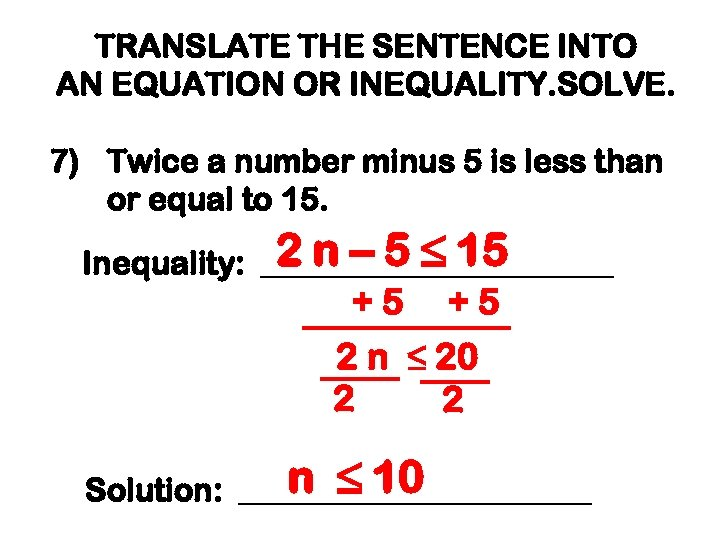TRANSLATE THE SENTENCE INTO AN EQUATION OR INEQUALITY. SOLVE. 7) Twice a number minus 5 is less than or equal to 15. 2 n – 5 ≤ 15 Inequality: ___________ +5 +5 2 n ≤ 20 2 2 n ≤ 10 Solution: ___________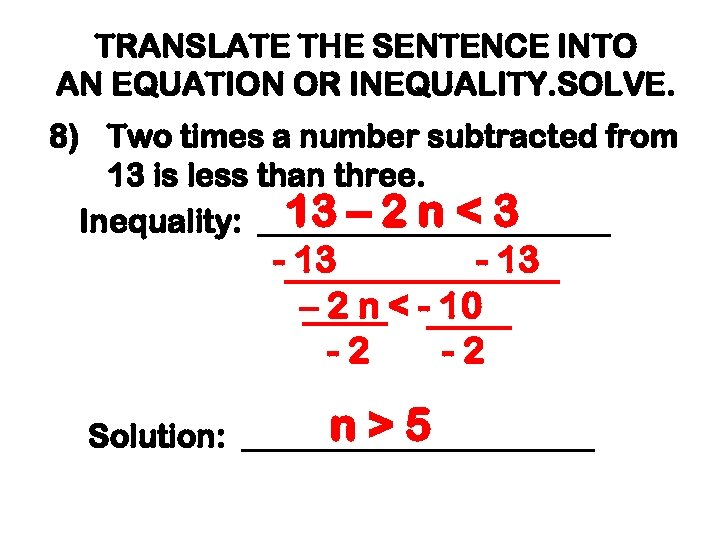TRANSLATE THE SENTENCE INTO AN EQUATION OR INEQUALITY. SOLVE. 8) Two times a number subtracted from 13 is less than three. 13 – 2 n < 3 Inequality: ___________ - 13 – 2 n < - 10 -2 -2 n>5 Solution: ___________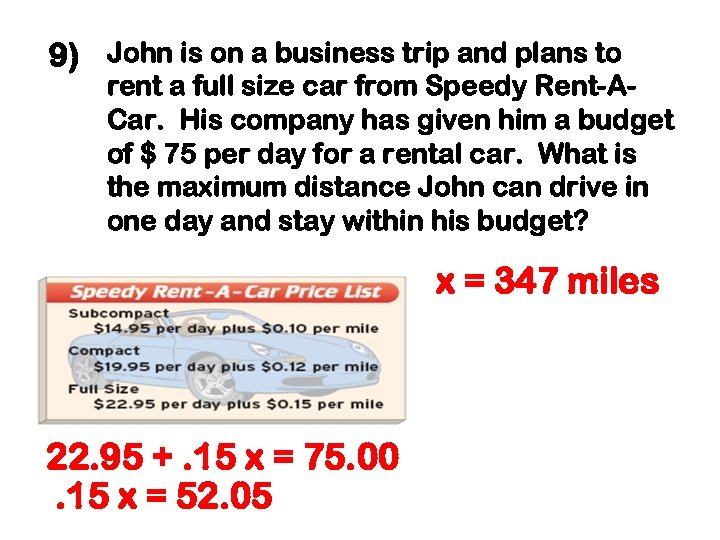9) John is on a business trip and plans to rent a full size car from Speedy Rent-ACar. His company has given him a budget of \$ 75 per day for a rental car. What is the maximum distance John can drive in one day and stay within his budget? x = 347 miles 22. 95 +. 15 x = 75. 00. 15 x = 52. 05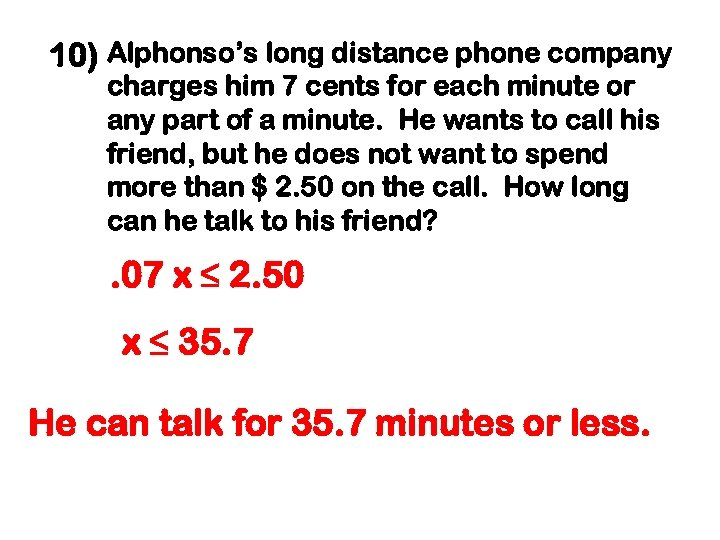10) Alphonso’s long distance phone company charges him 7 cents for each minute or any part of a minute. He wants to call his friend, but he does not want to spend more than \$ 2. 50 on the call. How long can he talk to his friend? . 07 x ≤ 2. 50 x ≤ 35. 7 He can talk for 35. 7 minutes or less.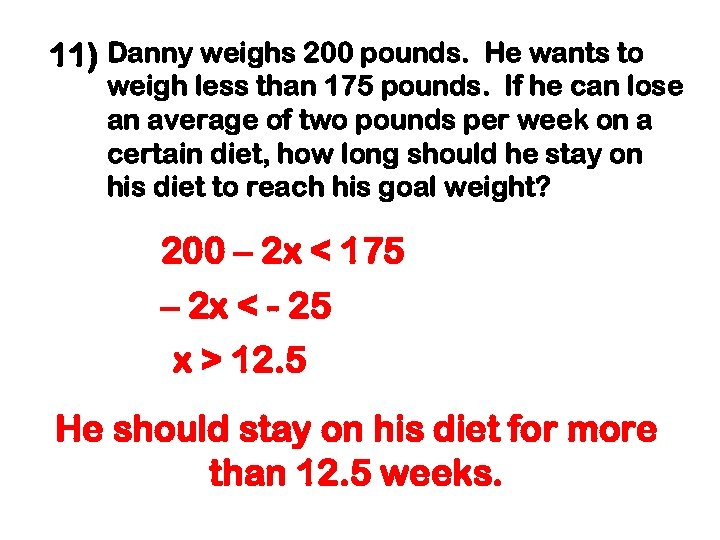11) Danny weighs 200 pounds. He wants to weigh less than 175 pounds. If he can lose an average of two pounds per week on a certain diet, how long should he stay on his diet to reach his goal weight? 200 – 2 x < 175 – 2 x < - 25 x > 12. 5 He should stay on his diet for more than 12. 5 weeks.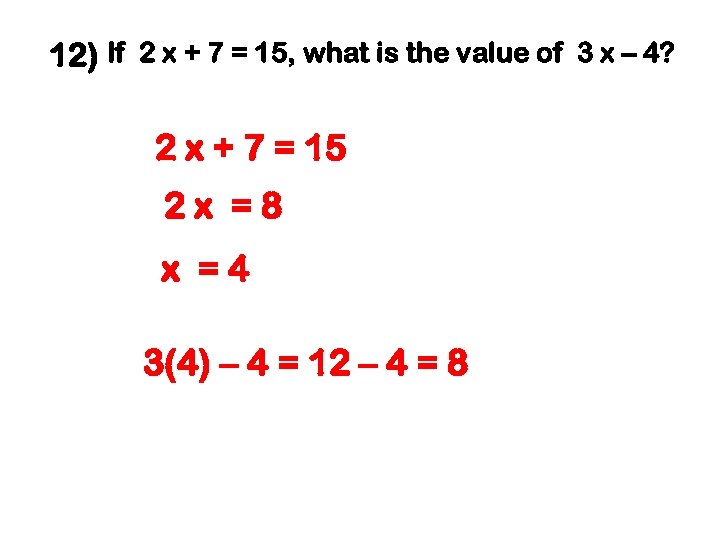12) If 2 x + 7 = 15, what is the value of 3 x – 4? 2 x + 7 = 15 2 x =8 x =4 3(4) – 4 = 12 – 4 = 8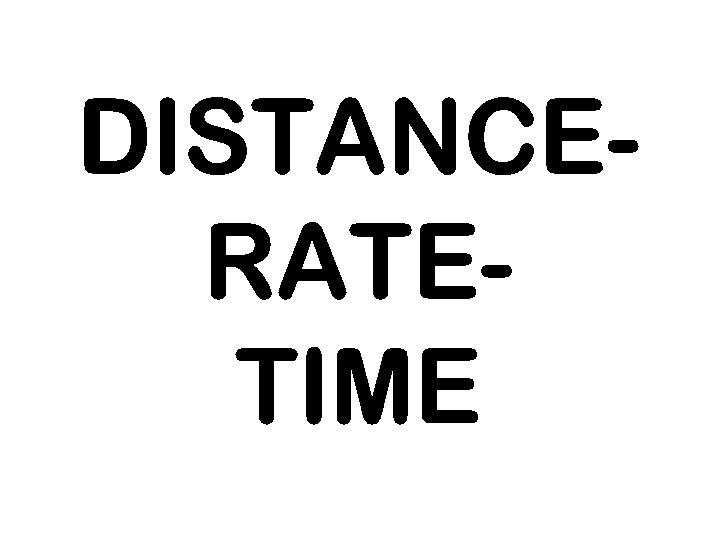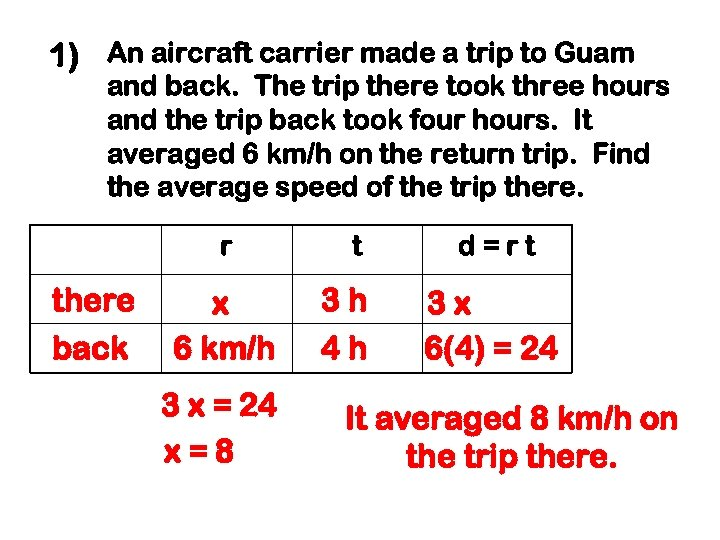1) An aircraft carrier made a trip to Guam and back. The trip there took three hours and the trip back took four hours. It averaged 6 km/h on the return trip. Find the average speed of the trip there. r there back x 6 km/h 3 x = 24 x=8 t 3 h 4 h d=rt 3 x 6(4) = 24 It averaged 8 km/h on the trip there.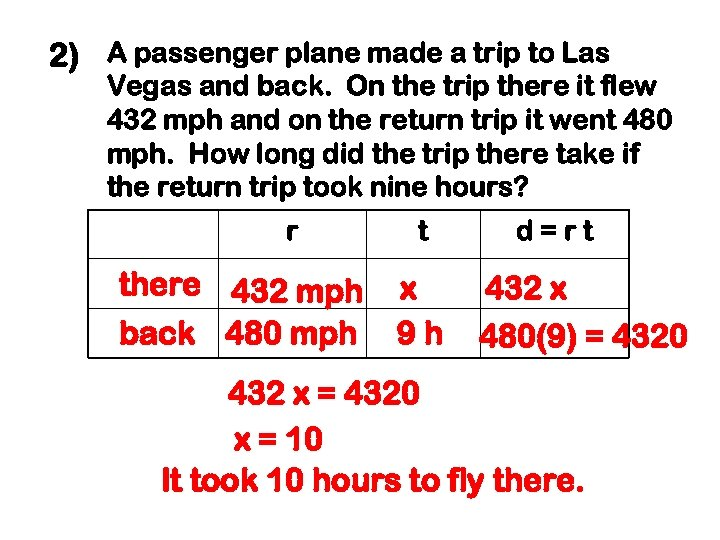2) A passenger plane made a trip to Las Vegas and back. On the trip there it flew 432 mph and on the return trip it went 480 mph. How long did the trip there take if the return trip took nine hours? r there 432 mph back 480 mph t x 9 h d=rt 432 x 480(9) = 4320 432 x = 4320 x = 10 It took 10 hours to fly there.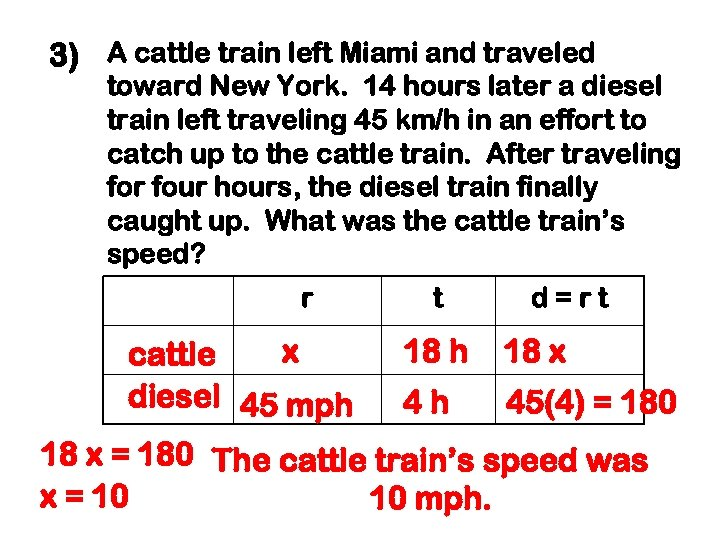3) A cattle train left Miami and traveled toward New York. 14 hours later a diesel train left traveling 45 km/h in an effort to catch up to the cattle train. After traveling for four hours, the diesel train finally caught up. What was the cattle train’s speed? r x cattle diesel 45 mph t d=rt 18 h 18 x 4 h 45(4) = 180 18 x = 180 The cattle train’s speed was x = 10 10 mph.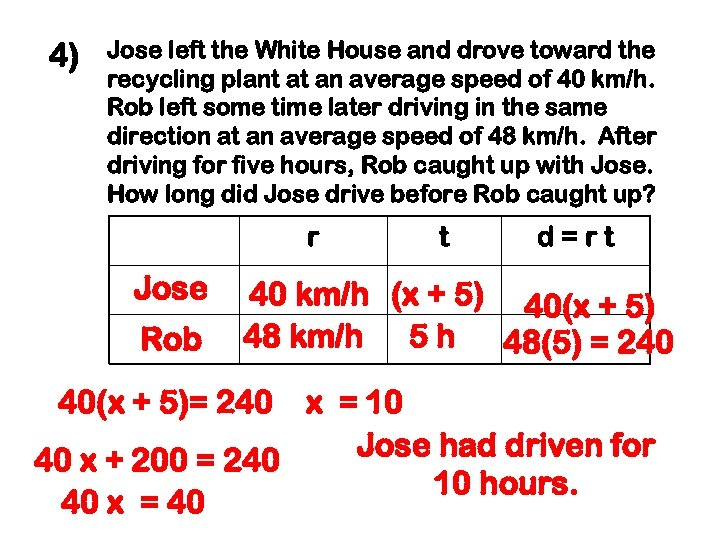4) Jose left the White House and drove toward the recycling plant at an average speed of 40 km/h. Rob left some time later driving in the same direction at an average speed of 48 km/h. After driving for five hours, Rob caught up with Jose. How long did Jose drive before Rob caught up? r Jose Rob t d=rt 40 km/h (x + 5) 40(x + 5) 48 km/h 5 h 48(5) = 240 40(x + 5)= 240 x = 10 Jose had driven for 40 x + 200 = 240 10 hours. 40 x = 40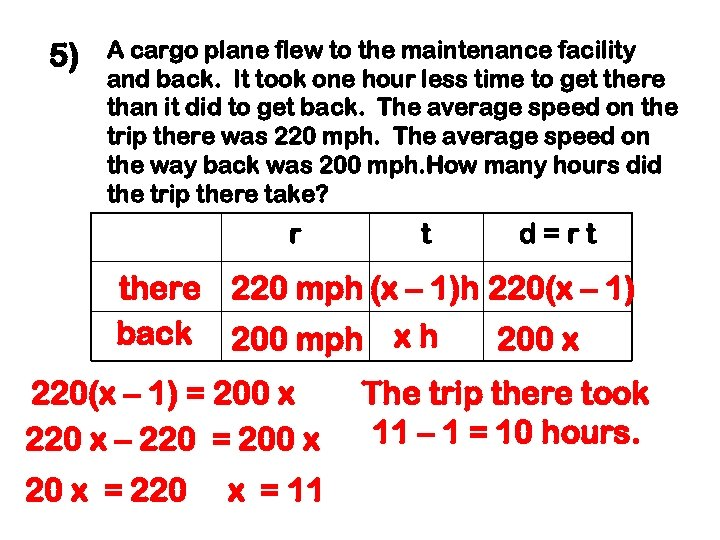5) A cargo plane flew to the maintenance facility and back. It took one hour less time to get there than it did to get back. The average speed on the trip there was 220 mph. The average speed on the way back was 200 mph. How many hours did the trip there take? r t d=rt there 220 mph (x – 1)h 220(x – 1) back 200 mph x h 200 x 220(x – 1) = 200 x 220 x – 220 = 200 x 20 x = 220 x = 11 The trip there took 11 – 1 = 10 hours.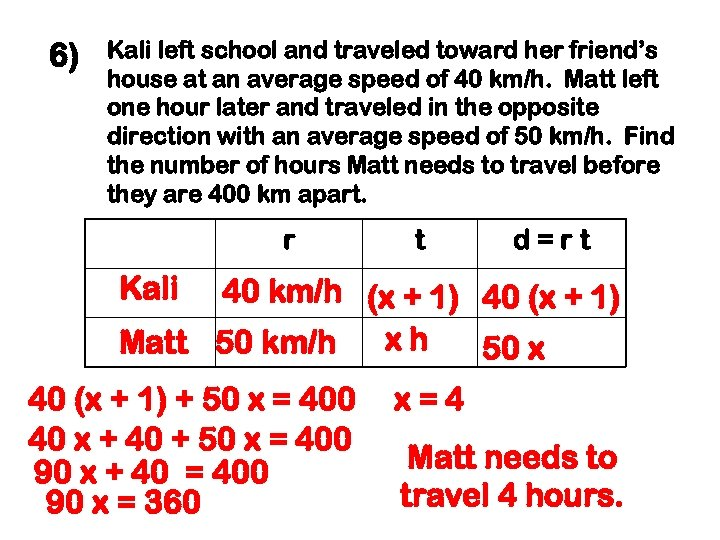6) Kali left school and traveled toward her friend’s house at an average speed of 40 km/h. Matt left one hour later and traveled in the opposite direction with an average speed of 50 km/h. Find the number of hours Matt needs to travel before they are 400 km apart. r t d=rt Kali 40 km/h (x + 1) 40 (x + 1) xh Matt 50 km/h 50 x 40 (x + 1) + 50 x = 400 40 x + 40 + 50 x = 400 90 x + 40 = 400 90 x = 360 x=4 Matt needs to travel 4 hours.